1597349100

# Big O Notation | time complexity of algorithms

Big O Notation explained in simple language, part of data structures and Algorithms series in JavaScript with examples .
Help me translate this video.

#algorithms

## Buddha Community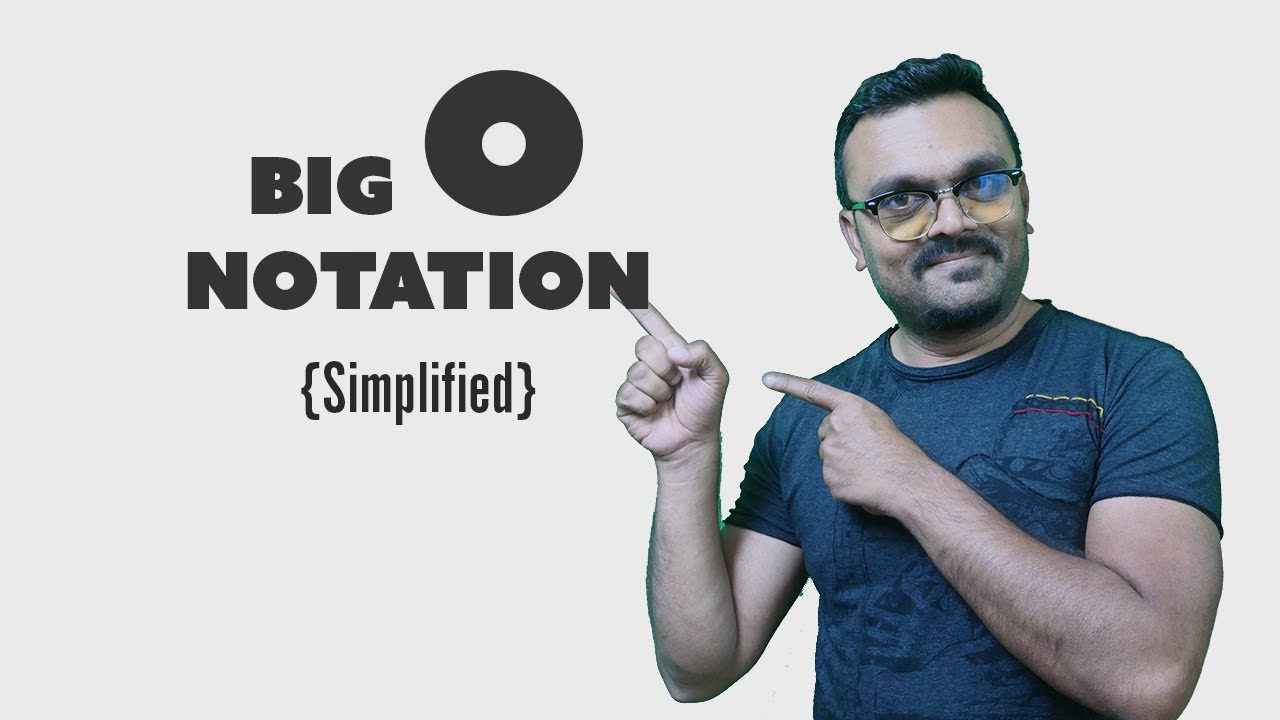1593442500

# Big O Notation and Time/Space Complexity

Big O notation is a commonly used metric used in computer science to classify algorithms based on their time and space complexity. The time and space here is not based on the actual number of operations performed or amount of memory used per se, but rather how the algorithm would scale with an increase or decrease in the amount of data in the input. The notation will represent how an algorithm will run in the worst-case scenario- what is the maximum time or space an algorithm could use? The complexity is written as O(x) where x is the growth rate of the algorithm in regards to n, which is the amount of data input. Throughout the rest of this blog, input will be referred to as n.

# O(1)

O(1) is known as constant complexity. What this implies is that the amount of time or memory does not scale with n at all. For time complexity, this means that n is not iterated on or recursed- generally a value will be selected and returned or a value with be operated on and returned.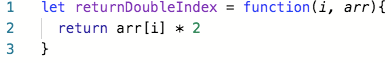Function that returns an index of an array doubled in O(1) time complexity.

For space, no data structures can be created that are a multiples of the size of n. Variables can be declared, but the number must not change with n.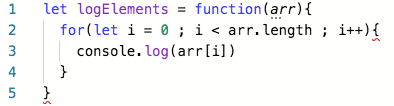Function that logs every element in an array with O(1) space.

# O(logn)

O(logn) is known as logarithmic complexity. The logarithm in O(logn) has a base of 2. The best way to wrap your head around this is to remember the concept of halving: every time n increases by an amount k, the time or space increases by k/2. There are several common algorithms that are O(logn) a vast majority of the time to keep an eye out for: binary search, searching for a term in a binary search tree, and adding items to a heap.

#algorithms #computer-science #big-o-notation #time-complexity #space-complexity #algorithms1594945860

## Big O Notation and Time Complexity

Big O notation is a simplified analysis of an algorithm’s efficiency. Big O notation gives us an algorithm’s complexity in terms of input size, N. It gives us a way to abstract the efficiency of our algorithm or code from the machines/computers they run on. We don’t care how powerful our machine is, but rather, the basic steps of the code. We can use big O to analyze both time and space. I will go over how we can use Big O to measure time complexity using Ruby for examples.

Types of measurement

There are a couple of ways to look at an algorithm’s efficiency. We can examine worst-case, best-case, and average-case. When we examine big O notation, we typically look at the worst-case. This isn’t to say the other cases aren’t as important.

General rules

1. Ignore constants

5n ->O(n)

Big O notation ignores constants. For example, if you have a function that has a running time of 5n, we say that this function runs on the order of the big O of N. This is because as N gets large, the 5 no longer matters.

2. In the same way that N grows, certain terms “dominate” others

Here’s a list:

O(1) < O(logn) < O(n) < O(nlogn) < O(n²) < O(2^n) < O(n!)

We ignore low-order terms when they are dominated by high order terms.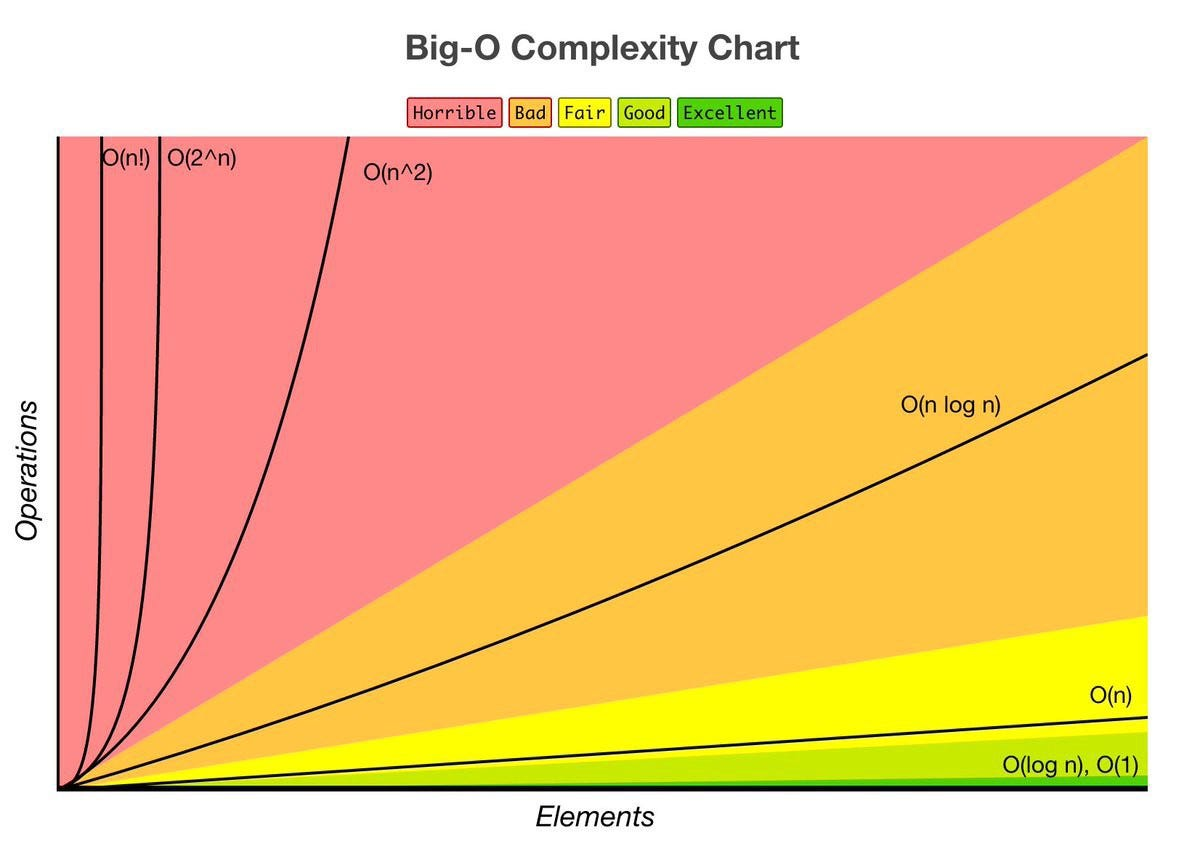Constant Time: O(1)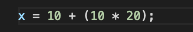This basic statement computes x and does not depend on the input size in any way. This is independent of input size, N. We say this is a “Big O of one” or constant time.

#time-complexity #algorithms #big-o-notation #flatiron-school #algorithms1594945560

## Big O Notation and Time Complexity

Big O notation is a simplified analysis of an algorithm’s efficiency. Big O notation gives us an algorithm’s complexity in terms of input size, N. It gives us a way to abstract the efficiency of our algorithm or code from the machines/computers they run on. We don’t care how powerful our machine is, but rather, the basic steps of the code. We can use big O to analyze both time and space. I will go over how we can use Big O to measure time complexity using Ruby for examples.

Types of measurement

There are a couple of ways to look at an algorithm’s efficiency. We can examine worst-case, best-case, and average-case. When we examine big O notation, we typically look at the worst-case. This isn’t to say the other cases aren’t as important.

General rules

1. Ignore constants

5n ->O(n)

Big O notation ignores constants. For example, if you have a function that has a running time of 5n, we say that this function runs on the order of the big O of N. This is because as N gets large, the 5 no longer matters.

2. In the same way that N grows, certain terms “dominate” others

Here’s a list:

O(1) < O(logn) < O(n) < O(nlogn) < O(n²) < O(2^n) < O(n!)

We ignore low-order terms when they are dominated by high order terms.Constant Time: O(1)This basic statement computes x and does not depend on the input size in any way. This is independent of input size, N. We say this is a “Big O of one” or constant time.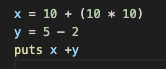total time = O(1) + O(1) + O(1) = O(1)

What happens when we have a sequence of statements? Notice that all of these are constant time. How do we compute big O for this block of code? We simply add each of their times and we get 3 * O(1). But remember we drop constants, so this is still big O of one.

Linear Time: O(n)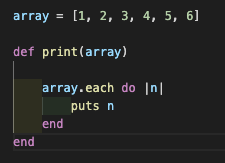The run time of this method is linear or O(n). We have a loop inside of this method which iterates over the array and outputs the element. The number of operations this loop performs will change depending on the size of the array. For example, an array of size 6 will only take 6 iterations, while an array of 18 elements would take 3 times as long. As the input size increases, so will the runtime.Big O notation is a simplified analysis of an algorithm’s efficiency. Big O notation gives us an algorithm’s complexity in terms of input size, N. It gives us a way to abstract the efficiency of our algorithm or code from the machines/computers they run on. We don’t care how powerful our machine is, but rather, the basic steps of the code. We can use big O to analyze both time and space. I will go over how we can use Big O to measure time complexity using Ruby for examples.

Types of measurement

There are a couple of ways to look at an algorithm’s efficiency. We can examine worst-case, best-case, and average-case. When we examine big O notation, we typically look at the worst-case. This isn’t to say the other cases aren’t as important.

General rules

#time-complexity #algorithms #big-o-notation #flatiron-school #algorithms1595543940

## Big O Notation: Calculating Time Complexity

Preparing for technical interviews can be difficult, frustrating, and confusing at times. As I continue my job search I thought I would take some time to share what I’ve learned thus far about calculating time complexity, and Big O notation. This topic wasn’t easy for me to grasp when it was first introduced at Flatiron, but the idea behind Big O is actually fairly simple once you delve into it a little further. Here is a rundown of how it works, and what you need to know to start nailing those tech interview questions!

First off, when people say “Big O” they’re really referring to Big ϴ (“big theta”). This concept looks to evaluate the limiting behavior of a function as it approaches a specific value/infinity. Essentially you’re looking at the worst case scenario when working with Big O or the “upper asymptotic bound.” To calculate time complexity you only need to look at the term with the largest exponent, ignore coefficients/smaller terms, and you can also count the number of nested loops in your function to help determine Big O. Let me give an example using Linear search with a Ruby implementation. In this example I’m trying to find a specific target number — 23 in a shuffled array of 60 numbers.

``````array = [*1..60].shuffle

def linear_search(array, target)
counter = 0

# iterate through the given array starting
# at index 0 and continue until the end

while counter < array.length
if array[counter] == target
# exit the loop if the target element is found
return "Took: #{counter} iterations to find the target"
else
counter += 1
end
end

return "#{target} could not be found in the array"
end
linear_search(array, 23)
``````

Running my method `linear_search` 5 times gave this output when trying to find the target — 23.

``````=> "Took: 12 iterations to find the target"
=> "Took: 20 iterations to find the target"
=> "Took: 37 iterations to find the target"
=> "Took: 55 iterations to find the target"
=> "Took: 30 iterations to find the target"
``````

Essentially what this output is telling us is that given our function the smallest number of iterations it could take to find the target would be 1, if it was shuffled to index 0 of the array. More importantly the worst case scenario would be 60 iterations if it got shuffled to the end. If our array was 150 numbers/items it would have a worst case scenario of 150 iterations. The Big O notation for Linear search is O(n), where n simply equals the number of elements in the collection.

#time-complexity #big-o-notation #data-structures #algorithms #algorithms1625640780

## Time Complexity & Big O notation | DSA-One Course - All you Need in One place

Hey guys, In this video, we’ll be talking about Time complexity and Big O notation. This is the first video of our DSA-One Course. We’ll also learn how to find the time complexity of Recursive problems.